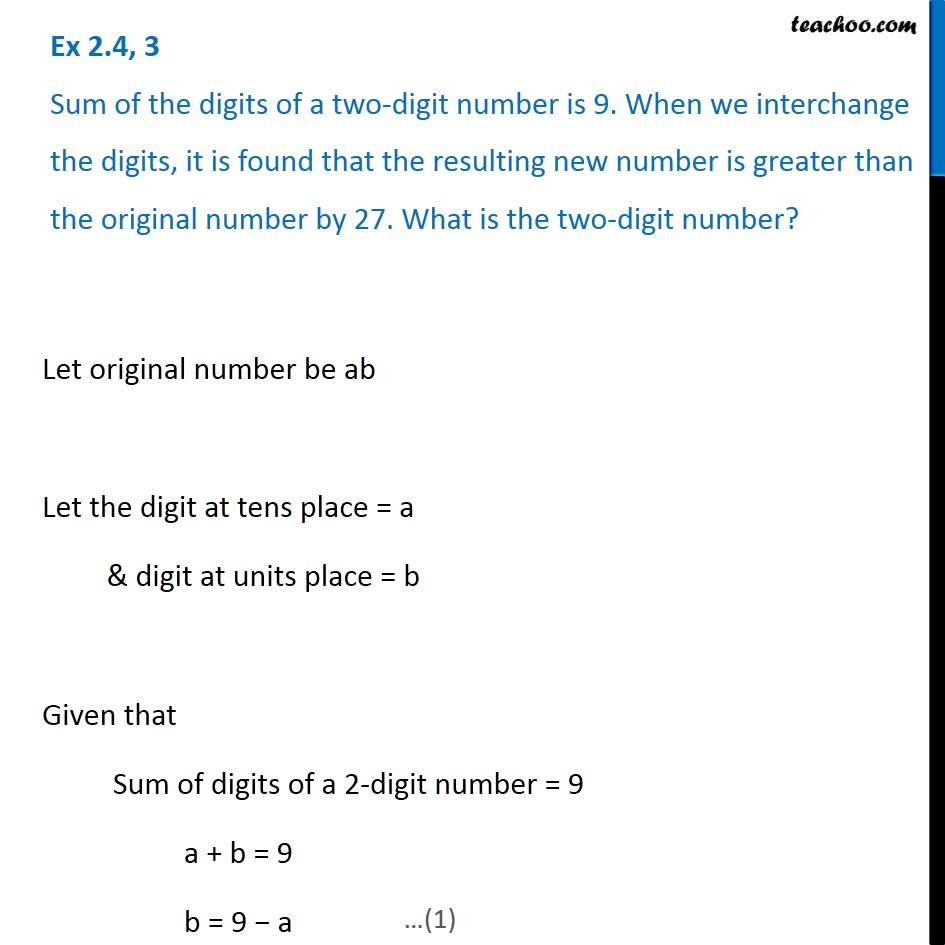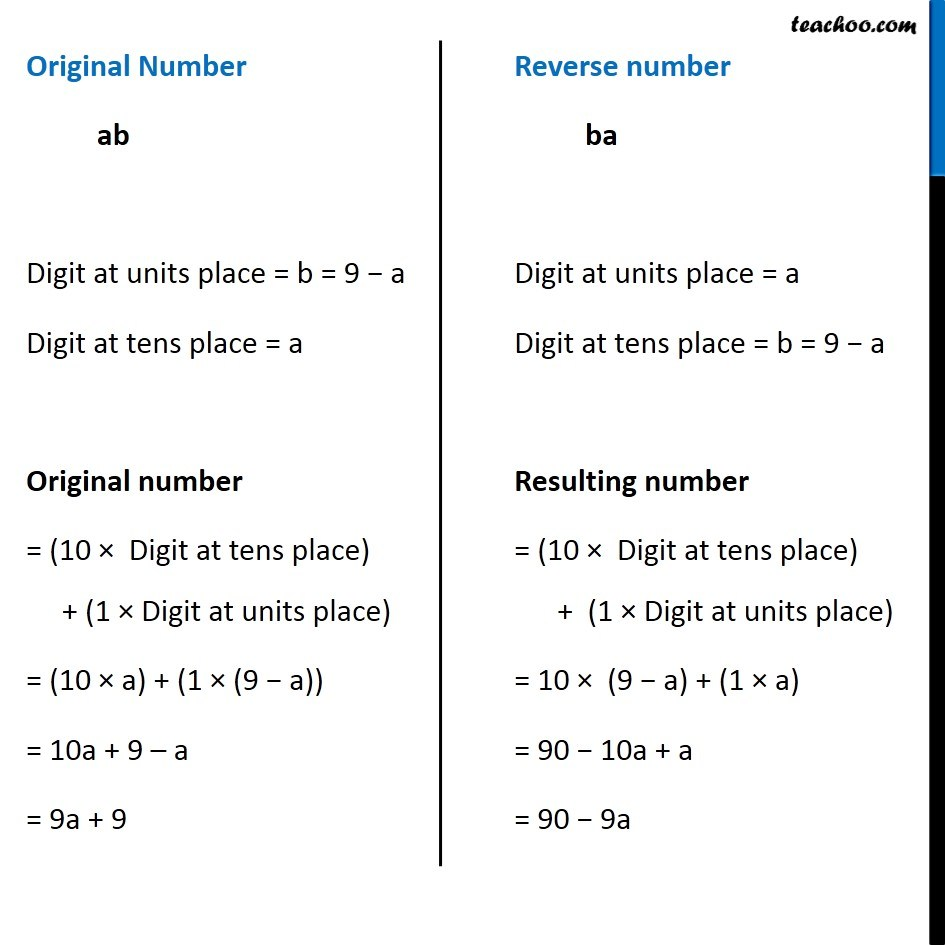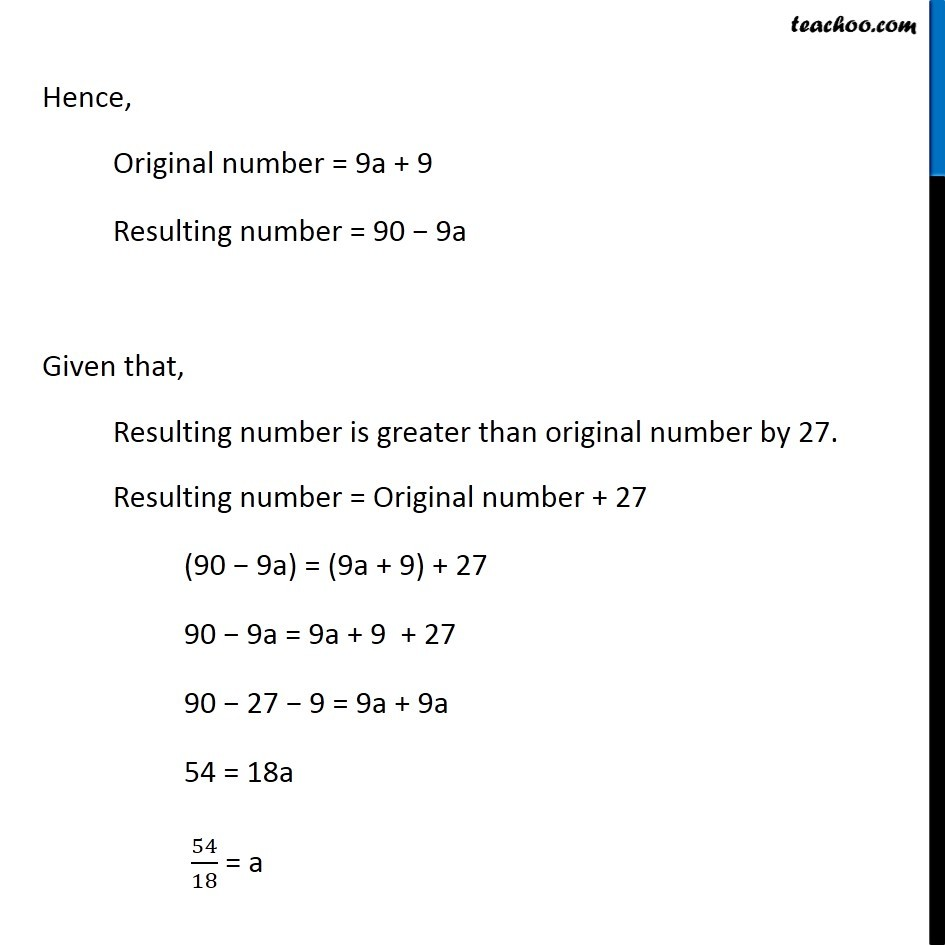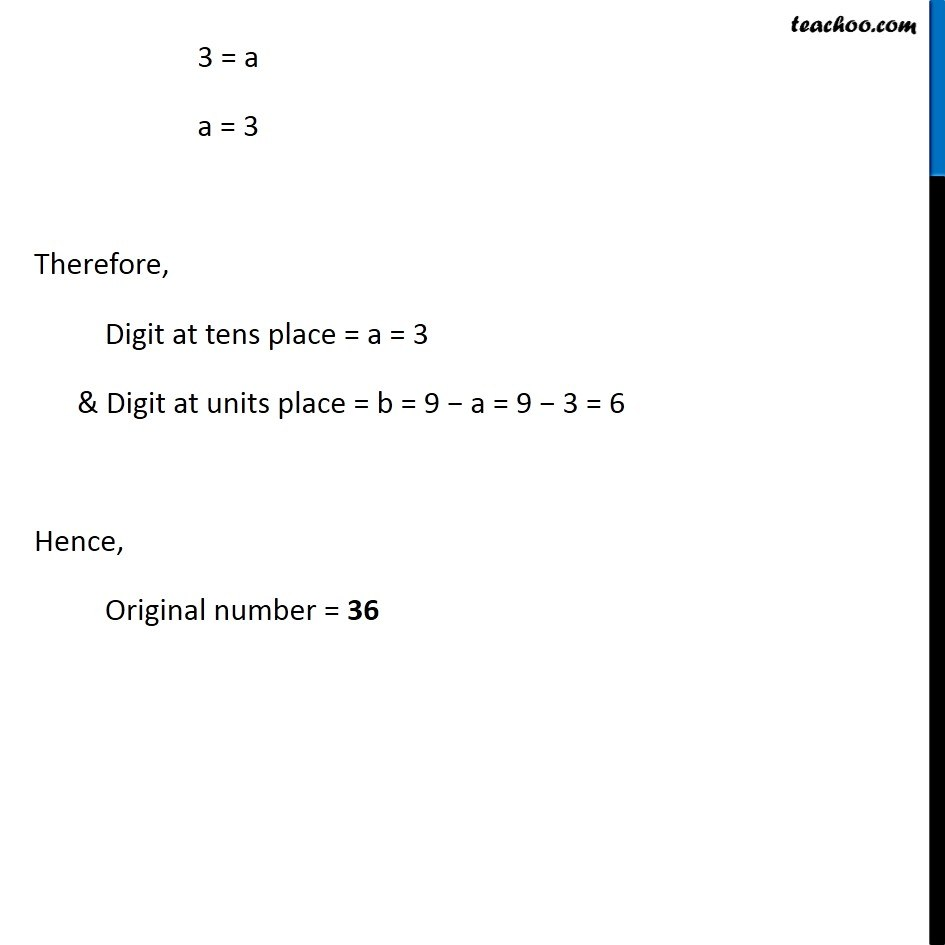To do this question, first check Two digit number and its reverse .1. Chapter 2 Class 8 Linear Equations in One Variable
2. Concept wise
3. Forming Linear Equations - Two digit number

Transcript

Ex 2.4, 3 Sum of the digits of a two-digit number is 9. When we interchange the digits, it is found that the resulting new number is greater than the original number by 27. What is the two-digit number?Let original number be ab Let the digit at tens place = a & digit at units place = b Given that Sum of digits of a 2-digit number = 9 a + b = 9 b = 9 a Original Number ab Digit at units place = b = 9 a Digit at tens place = a Original number = (10 Digit at tens place) + (1 Digit at units place) = (10 a) + (1 (9 a)) = 10a + 9 a = 9a + 9 Reverse number ba Digit at units place = a Digit at tens place = b = 9 a Resulting number = (10 Digit at tens place) + (1 Digit at units place) = 10 (9 a) + (1 a) = 90 10a + a = 90 9a Hence, Original number = 9a + 9 Resulting number = 90 9a Given that, Resulting number is greater than original number by 27. Resulting number = Original number + 27 (90 9a) = (9a + 9) + 27 90 9a = 9a + 9 + 27 90 27 9 = 9a + 9a 54 = 18a 54/18 = a 3 = a a = 3 Therefore, Digit at tens place = a = 3 & Digit at units place = b = 9 a = 9 3 = 6 Hence, Original number = 36